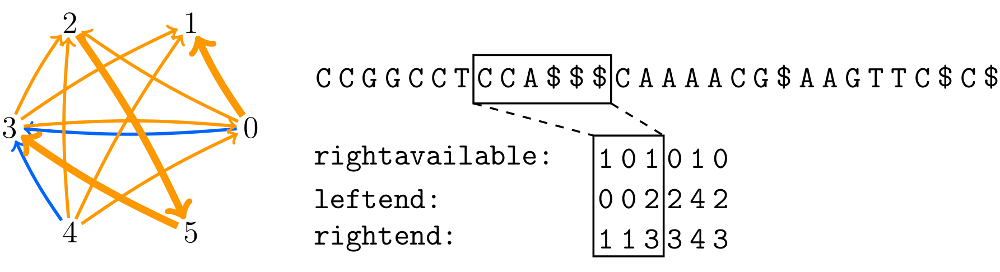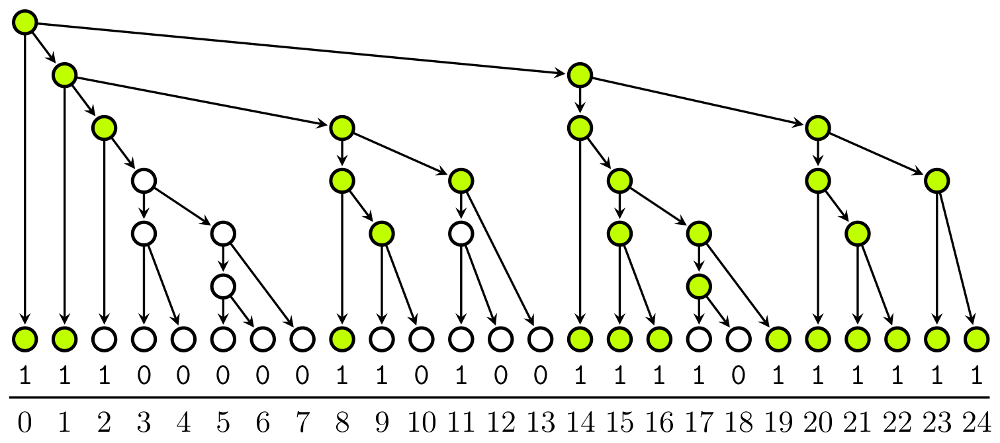## Who am I

I am a postdoctoral researcher in computer science and bioinformatics. Below is a list of some older projects of mine (who has time to maintain an up-to-date personal website, anyway?). For more recent work, check out my Google Scholar page. For notes relating to research, see my research blog. I also have some writings unrelated to my research at my regular blog. I'm also an amateur musician publishing tunes on Youtube under the alias Bytenommer.

## Research Projects

### Themisto: A pseudoaligner for metagenomics based on a succinct colored de Bruijn graphA metanenomic sample is a set of sequences of reads from microbial life living in a particular environment. Standard analysis involves estimating the species composition of the environment by aligning the reads against a reference database. Since the age of pangenomics, alignment is preferentially done against a variation graph encompassing all variation within a species.

Themisto is a space-efficient tool for indexing such variation graphs. The Themisto index is a compressed colored de-bruijn graph of order $k$, where each node has a set of colors representing the reference sequences that contain the $k$-mer (substring of length $k$) corresponding to the node. Reads are pseudoaligned to the index using a method similar to the one used by the tool Kallisto: all $k$-mers of the read are located in the de-bruijn graph and the intersection of the color sets of the nodes is returned.

Github Website

### Shortest common superstring approximation in compact working spaceThe shortest common superstring problem is a classical problem in string processing. It is defined as follows. Let $\mathcal S = S_1,\ldots,S_m$ be a set of strings from an alphabet $\Sigma$ of size $\sigma$. The problem is to find the shortest string that contains every string in $\mathcal S$ as a substring. If there are multiple solutions, it is enough to return one. We have a solution that computer a greedy approximation in $O(n \log \sigma)$ bits of working space.

Github Paper

### Variable-order Markov models in compact spaceMarkov models are a basic tool in biological sequence analysis. They model sequences as random processes following simple rules where the key assumption is that the process has limited memory, i.e. the distribution of the next symbol in the sequence depends only on a few of the previous symbols. This is called the Markov property. The previous symbols that determine the distribution of the next symbol are called the current state or the current context of the process. The model is composed of a list of transition probabilities between all possible states. The transition probabilities are usually learned automatically from training data.

In fixed-order models, the state of the chain is the last $k$ characters of the current sequence, for some constant $k$. In variable-order models, the state can have variable length. We have implemented and designed a space-efficient framework for representing and running large variable-order Markov models.

Github Paper

### Space-efficient clustering of metagenomic reads

We present a framework for implementing a clustering pipeline for metagenomic reads. The pipeline works in two parts. First, reads with long shared $k$-mers are grouped together and second, groups sharing similar sequence statistics are merged.

Github Paper

## Hobby Projects

### Finnish language article generation with an LSTM neural network

I trained a textgenrnn-LSTM neural network that wrote an article to the Finnish computer culture magazine Skrolli. The article was published along with a 5-page writeup on how it was done.

Published in: Skrolli-magazine issue 2019.1. (Finnish)

### Real time graphics demo using raymarching

A 3d-graphics demo for Skrolli party 2019.

Writeup of the techniques used: Skrolli magazine issue 2019.3 (Finnish).

### Basic 3d-graphics engine

A 3d-graphics demo for Assembly 2018.

Writeup of the techniques used: Skrolli magazine issue 2018.3 (Finnish).